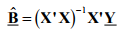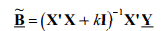# Ridge Regression: Simple Definition

Ridge regression is a way to create a parsimonious model when the number of predictor variables in a set exceeds the number of observations, or when a data set has multicollinearity (correlations between predictor variables).

Tikhivov’s method is basically the same as ridge regression, except that Tikhonov’s has a larger set. It can produce solutions even when your data set contains a lot of statistical noise (unexplained variation in a sample).

## Ridge Regression vs. Least Squares

Least squares regression isn’t defined at all when the number of predictors exceeds the number of observations; It doesn’t differentiate “important” from “less-important” predictors in a model, so it includes all of them. This leads to overfitting a model and failure to find unique solutions. Least squares also has issues dealing with multicollinearity in data. Ridge regression avoids all of these problems. It works in part because it doesn’t require unbiased estimators; While least squares produces unbiased estimates, variances can be so large that they may be wholly inaccurate. Ridge regression adds just enough bias to make the estimates reasonably reliable approximations to true population values.

## Shrinkage

Ridge regression uses a type of shrinkage estimator called a ridge estimator. Shrinkage estimators theoretically produce new estimators that are shrunk closer to the “true” population parameters. The ridge estimator is especially good at improving the least-squares estimate when multicollinearity is present.

## Regularization

Ridge regression belongs a class of regression tools that use L2 regularization. The other type of regularization, L1 regularization, limits the size of the coefficients by adding an L1 penalty equal to the absolute value of the magnitude of coefficients. This sometimes results in the elimination of some coefficients altogether, which can yield sparse models. L2 regularization adds an L2 penalty, which equals the square of the magnitude of coefficients. All coefficients are shrunk by the same factor (so none are eliminated). Unlike L1 regularization, L2 will not result in sparse models.

A tuning parameter (λ) controls the strength of the penalty term. When λ = 0, ridge regression equals least squares regression. If λ = ∞, all coefficients are shrunk to zero. The ideal penalty is therefore somewhere in between 0 and ∞.

## On Mathematics

OLS regression uses the following formula to estimate coefficients:If X is a centered and scaled matrix, the crossproduct matrix (X`X) is nearly singular when the X-columns are highly correlated. Ridge regression adds a ridge parameter (k), of the identity matrix to the cross product matrix, forming a new matrix (X`X + kI). It’s called ridge regression because the diagonal of ones in the correlation matrix can be described as a ridge. The new formula is used to find the coefficients:Choosing a value for k is not a simple task, which is perhaps one major reason why ridge regression isn’t used as much as least squares or logistic regression. You can read one way to find k in Dorugade and D. N. Kashid’s paper Alternative Method for Choosing Ridge Parameter for Regression..

For a more rigorous explanation of the mechanics behind the procedure, you may want to read Wessel N. van Wieringen’s Ridge Regression Lecture Notes.

References:
Chatterjee, S. & Hadi, A. (2006). Regression Analysis by Example. Wiley.
Dorugade and D. N. Kashid. Alternative Method for Choosing Ridge Parameter for Regression. Applied Mathematical Sciences, Vol. 4, 2010, no. 9, 447 – 456. Retrieved July 29, 2017 from: http://www.m-hikari.com/ams/ams-2010/ams-9-12-2010/dorugadeAMS9-12-2010.pdf.
Wessel N. van Wieringen. Lecture notes on RR. Retrieved July 29, 2017 from: https://arxiv.org/pdf/1509.09169.pdf

CITE THIS AS:
Stephanie Glen. "Ridge Regression: Simple Definition" From StatisticsHowTo.com: Elementary Statistics for the rest of us! https://www.statisticshowto.com/ridge-regression/